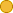## ← 01-03 Total Probability

• 2 Followers
• 66 Lines

dummy description

### Get Embed Code x Embed video Use the following code to embed this video. See our usage guide for more details on embedding. Paste this in your document somewhere (closest to the closing body tag is preferable): ```<script type="text/javascript" src='https://amara.org/embedder-iframe'></script> ``` Paste this inside your HTML body, where you want to include the widget: ```<div class="amara-embed" data-url="http://www.youtube.com/watch?v=n1EacrqyCs8" data-team="udacity"></div> ``` 11 Languages

•English [en] original
•Bengali [bn] incomplete
•Spanish [es]
•Italian [it]
•Japanese [ja]
•Korean [ko] incomplete
•Polish [pl] incomplete
•Portuguese [pt]
•Russian [ru]
•Vietnamese [vi] incomplete
•Chinese, Simplified [zh-cn] incomplete

Showing Revision 2 created 04/11/2012 by Anna Chiara Bellini.

1. Let me begin my story in a world where our robot resides.
2. Let's assume the robot has no clue where it is.
3. Then we would model this with a function--I'm going to draw into this diagram over here
4. where the vertical axis is the probability for any location in this world,
5. and the horizontal axis corresponds to all the places in this 1-dimensional world.
6. The way I'm going to model the robot's current belief about where it might be,
7. it's confusion is by a uniform function that assigns equal weight
8. to every possible place in this world.
9. That is the state of maximum confusion
10. Now, to localize the world has to have some distinctive features.
11. Let's assume there are 3 different landmarks in the world.
12. There is a door over here, there's a door over here, and a 3rd one way back here.
13. For the sake of the argument,
14. let's assume they all look alike, so they're not distinguishable,
15. but you can distinguish the door from the non-door area--from the wall.
16. Now let's see how the robot can localize itself by assuming it senses,
17. and it senses that it's standing right next to a door.
18. So all it knows now is that it is located, likely, next to a door.
19. How would this affect our belief?
20. Here is the critical step for localization.
21. If you understand this step, you understand localization.
22. The measurement of a door transforms our belief function,
23. defined over possible locations, to a new function that looks pretty much like this.
24. For the 3 locations adjacent to doors, we now have an increased belief of being there
25. whereas all the other locations have a decreased belief.
26. This is a probability distribution that assigns higher probability for being next to a door,
27. and it's called the posterior belief where the word "posterior" means it's after a measurement has been taken.
28. Now, the key aspect of this belief is that we still don't know where we are.
29. There are 3 possible door locations, and in fact, it might be
30. that the sensors were erroneous, and we accidentally saw a door where there's none.
31. So there is still a residual probability of being in these places over here,
32. but these three bumps together really express our current best belief of where we are.
33. This representation is absolutely core to probability and to mobile robot localization.
34. Now let's assume the robot moves.
35. Say it moves to the right by a certain distance.
36. Then we can shift the belief according to the motion.
37. And the way this might look like is about like this.
38. So this bump over here made it to here.
39. This guy went over here, and this guy over here.
40. Obviously, this robot knows its heading direction.
41. It's moving to the right in this example,
42. and it knows roughly how far it moved.
43. Now, robot motion is somewhat uncertain.
44. We can never be certain where the robot moved.
45. So these things are a little bit flatter than these guys over here.
46. The process of moving those beliefs to the right side is technically called a convolution.
47. Let's now assume the robot senses again, and for the sake of the argument,
48. let's assume it sees itself right next to a door again,
49. so the measurement is the same as before.
50. Now the most amazing thing happens.
51. We end up multiplying our belief, which is now prior to the second measurement,
52. with a function that looks very much like this one over here,
53. which has a peak at each door and out comes a belief that looks like the following.
54. There are a couple of minor bumps, but the only really big bump is this one over here.
55. This one corresponds to this guy over there in the prior,
56. and it's the only place in this prior that really corresponds to the measurement of a door,
57. whereas all the other places of doors have a low prior belief.
58. As a result, this function is really interesting.
59. It's a distribution that focuses most of its weight
60. onto the correct hypothesis of the robot being in the second door,
61. and it provides very little belief to places far away from doors.
62. At this point, our robot has localized itself.
63. If you understood this, you understand probability, and you understand localization.
64. So congratulations. You understand probability and localization.
65. You might not know yet, but that's really a core aspect of understanding
66. a whole bunch of things I'm going to teach you in the class today.